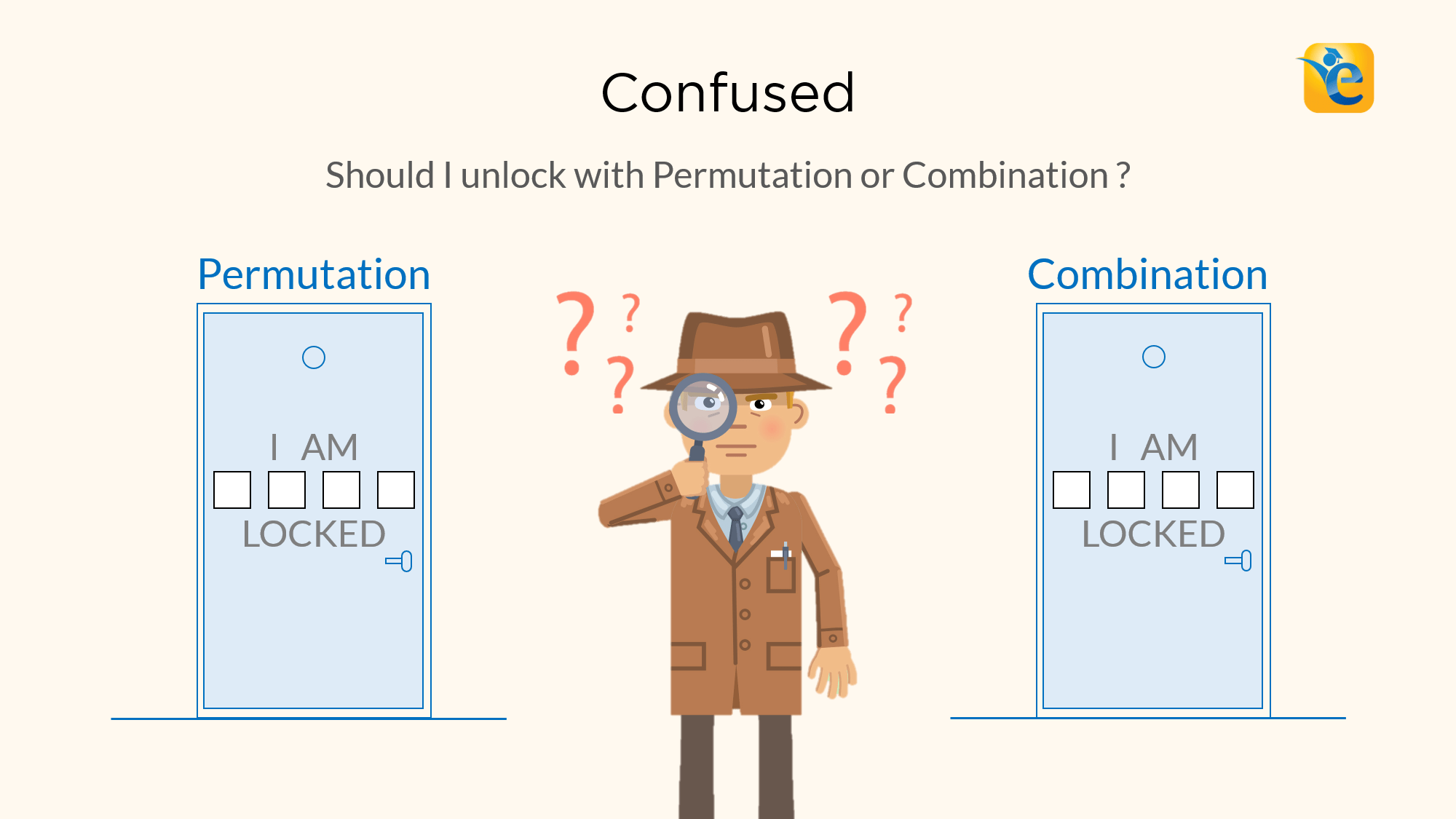# Difference between Permutation and Combination explained with examples

Permutations and Combinations is a topic full of conundrums. The biggest one is, understanding the difference between permutation and combination. Should I solve this question using permutation or combination? In this article, we will give you a foolproof method to differentiate between the two. In the last article of ‘Permutation and Combination’ series we talked

Read More# How to use Tree Structures to solve GMAT arithmetic problems

Read More# P is a particle on the circle shown above. What is the length of the path travelled by P in one complete revolution around the circle? – OGQR 2020 Question #212 with Solution

Solution Steps 1 & 2: Understand Question and Draw Inferences We are given: P is a particle on the circle, as shown in the given diagram. We need to find the length of the path travelled by P in one complete revolution around the circle. In one complete revolution, P will cover the entire length

Read More# What was the population of City X in 2002? – OGQR 2020 Question #209 with Solution

Solution Steps 1 & 2: Understand Question and Draw Inferences In this question, we are asked to find the population of city X in 2002. We do not have much information to infer from, let us move to analyse the statements.   Step 3: Analyse Statement 1 “X’s population in 2002 increased by 2 percent, or

Read More# Carl averaged 2m miles per hour on a trip that took him h hours. If Ruth made the same trip in h hours, what was her average speed in miles per hour? – OGQR 2020 Question #82 with Solution

Solution Given In this question, we are given Carl averaged 2m miles per hour on a trip that took him h hours. Ruth made the same trip in (2/3)h hours To Find We need to determine The average speed of Ruth, in miles per hour. Approach & Working Both of them are making the same

Read More# If Car A took n hours to travel 2 miles and Car B took m hours to travel 3 miles, which of the following expresses the time it would take Car C, traveling at the average (arithmetic mean) of those rates, to travel 5 miles? – OGQR 2020 Question #79 with Solution

Solution Given In this question, we are given Car A took n hours to travel 2 miles. Car B took m hours to travel 3 miles. Car C travels at the average (arithmetic mean) of the travel rates of A and B. To Find We need to determine The time car C would have taken

Read More# A paint mixture was formed by mixing exactly 3 colors of paint. By volume, the mixture was x% blue paint, y% green paint, and z% red paint. If exactly 1 gallon of blue paint and 3 gallons of red paint were used, how many gallons of green paint were used? – OG 2020 Question #346 with Solution

Solution Steps 1 & 2: Understand Question and Draw Inferences In this question, we are given A paint mixture was formed by mixing exactly 3 colors of paint. By volume, the mixture was x% blue paint, y% green paint, and z% red paint. Exactly 1 gallon of blue paint and 3 gallons of red paint

Read More# A company produces a certain toy in only 2 sizes, small or large, and in only 2 colors, red or green. If, for each size, there are equal numbers of red and green toys in a certain production lot, what fraction of the total number of green toys is large? – OG 2020 Question #323 with Solution

Solution Steps 1 & 2: Understand Question and Draw Inferences In this question, we are given A company produces a certain toy in only 2 sizes, small or large, and in only 2 colors, red or green. For each size, there are equal numbers of red and green toys in a certain production lot. We

Read More# A certain painting job requires a mixture of yellow, green, and white paint. If 12 quarts of paint are needed for the job, how many quarts of green paint are needed? – OG 2020 Question #319 with Solution

Read MoreRead More